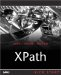Creating Comparison Expressions

Comparison expressions return the result of comparing two values. XPath 2.0 actually supports four kinds of comparison expressions: value comparisons, general comparisons, node comparisons, and order comparisons.

We'll take a look at them all here, starting with value and general comparisons. These two types of comparisons have been added so that XPath 2.0 can support comparisons with both single values and with sequences.

Value Comparisons

You use the value comparison operators when you're working with atomic values. Here they are:

• eq Equals

• ne Not equals

• lt Less than

• le Less than or equal to

• gt Greater than

• ge Greater than or equal to

These operators give you a result of true or false . Here's an examplesay that \$temperature holds the value 68; in that case, this expression would evaluate to true :

\$temperature lt 72

This comparison is true only if \$planet has a single < name > child element and its value is "Venus":

\$planet/name eq "Venus"

SHOP TALK : DATA TYPES AND TYPE ERRORS

Here's something to noteif \$planet/name evaluates to more than one name node, or \$planet/name evaluates to one name node, which contains more than one validated string, a type error is raised.

It's important to realize that type errors are the most significant difference between XPath 1.0 and XPath 2.0. If the XPath processor determines that there's been a type error, even a relatively innocent one like this one, a type error occurs.

Strong typing like this is at the very heart of XPath 2.0. In my work with XPath 2.0, that is by far the biggest difference between XPath 1.0 and 2.0.

However, how type errors are handled is up to the implementation. So far, all that usually happens is that XPath or XQuery processors simply stop and display an error message.

That's not what you want to have happen when someone else is using your XPath or XQuery expressions, of course, so be careful. I always spend some time trying to think of type problems that could break XPath expressions, and try to make sure that such problems won't arise. Until XPath processors come up with some way of handling type errors as recoverable errors, the strong data typing in XPath 2.0 is something I recommend you pay special attention to.

General Comparisons

You can use general comparisons on sequences (including singleton sequences). Here are the general comparisons:

• = Equals

• != Not equals

• < Less than

• <= Less than or equal to

• > Greater than

• >= Greater than or equal to

ESCAPING RULES

As in XPath 1.0, when you use XPath expressions inside an XML document, the XML escaping rules for special characters should be followed. For example, "<" should be written as "&lt;".

You use these operators on sequences. (What actually happens is that a value comparison operator, eq , ne , lt , le , gt , or ge depending on which corresponding general comparison operator was usedis used to compare individual items in the sequence.) The software evaluating a general comparison usually will return a value of true as soon as it finds an item in the first operand and an item in the second operand for which the value comparison is true.

Here's an example pointing out how these operators deal with sequences, not just individual values. In this case, we're comparing two sequences, (1, 2) and (2, 3) with the general equality operator:

(1, 2) = (2, 3)

In this case, the result is true because the value 2 appears in both sequences.

Here, however, the result is false, because there is no value in the first sequence that is equal to a value in the second:

(1, 2) = (3, 4)

As with value comparisons, this comparison is true only if \$planet has a single <name> child element and its value is "Venus":

\$planet/name = "Venus"

Node Comparisons

You can use node comparison expressions to compare nodes using the is operator.

A comparison expression with the is operator is true if the two operands are nodes that are identical; otherwise it is false.

For example, this comparison is true only if the left and right sides each evaluate to exactly the same single node:

//planet[name="Venus"] is //planet[days=116.75]

Order Comparisons

You use order comparison expressions to compare the order of nodes; both operands here must be either a single node or an empty sequence (if either operand is an empty sequence, the result of the comparison is an empty sequence). Here are the order comparison operators:

• << Earlier than

• >> Later than

Using the << operator returns true if the first operand node is earlier than the second operand node in document order; otherwise it returns false . Using the >> operator returns true if the first operand node is later than the second operand node in document order; otherwise it returns false .

Here's an example:

//planet[name="Venus"] << //planet[days=116.75]

This example returns true if the node matched by //planet[name="Venus"] comes earlier in document order than the node matched by //planet[days=116.75] .XPath Kick Start: Navigating XML with XPath 1.0 and 2.0
ISBN: 0672324113
EAN: 2147483647
Year: 2002
Pages: 131

Similar book on Amazon